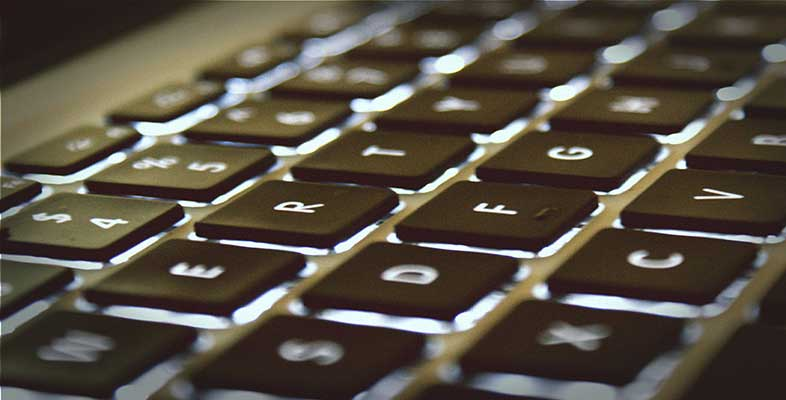Computers and computer systems

Start this free course now. Just create an account and sign in. Enrol and complete the course for a free statement of participation or digital badge if available.

Free course

# 4.2 Representing data

But if all the data and computer instructions within a computer are represented by 1s and 0s, how can this limited set of conditions be used to represent, for instance, every letter of the alphabet that might be typed into a computer from a keyboard? Activity 4 showed that there are four possible combinations of 1s and 0s in a 2-bit binary code. So if you had only two bits available you could only represent four different letters, e.g. ‘a’ could be represented by 00, ‘b’ by 01, ‘c’ by 10 and ‘d’ by 11. This shows that a 2-bit binary code can only represent four items of data.

## Activity 5 (Self assessment)

Write down all the possible combinations of a 3-bit binary code and state how many items of data three bits can represent.

### Answer

The possible combinations of a 3-bit binary code are 000, 001, 010, 011, 100, 101, 110, 111. Hence the three bits can represent 8 items of data.

Interestingly, a pattern can be deduced for the relationship between the number of bits and the number of items they represent.

• 2 bits can represent 2 × 2 = 22 = 4 items

• 3 bits can represent 2 × 2 × 2 = 23 = 8 items and following on with the same pattern

• 4 bits can represent 2 × 2 × 2 × 2 = 24 = 16 items.

Study note: If you are unsure of the use of the mathematical notation 22, 23 etc., you will find it helpful to refer to the Numeracy Resource below.

Click on the 'View document' link below to open the 'Numeracy resource'.

View document [Tip: hold Ctrl and click a link to open it in a new tab. (Hide tip)]

A group of eight bits is called a byte, so an 8-bit binary code is 1 byte long, a 16-bit binary code is 2 bytes long and a 64-bit binary code is 8 bytes long.

## Activity 6 (Self assessment)

1. How many bits are there in 4 bytes?

2. How many items of data can be represented by 1 byte?

### Answer

1. Four bytes contain 4 × 8 = 32 bits.

2. Since one byte contains 8 bits, the number of items that can be represented by one byte is 28 = 256. (Note that if you had to work out the number of items that eight bits could represent by writing down all possible combinations of 8 bits it would be very tedious and there would be a strong possibility of making an error. Using the calculation 28 = 256 is much easier way of finding the answer, as would be 216 = 65 536 for the number of combinations of a 16-bit binary code.)

In general, computers that perform more complex tasks at higher speeds use a larger number of bits to represent their data and instructions. The very simple central heating controller, which only has to do a limited amount of processing, may use an 8-bit representation. More powerful computers will use 16-, 32- or 64-bit representations.

When a computer is running a program a lot of data is being passed around the various elements within the system. The data received by the input subsystem(s) must be passed to the processor in a form it can use, and the processor in turn must present data to the output subsystem(s) in the required format. Even more fundamentally, the processor must be able to recognise each instruction within the program and execute it.

T224_1

### Take your learning further

Making the decision to study can be a big step, which is why you'll want a trusted University. The Open University has 50 years’ experience delivering flexible learning and 170,000 students are studying with us right now. Take a look at all Open University courses.

If you are new to University-level study, we offer two introductory routes to our qualifications. You could either choose to start with an Access module, or a module which allows you to count your previous learning towards an Open University qualification. Read our guide on Where to take your learning next for more information.

Not ready for formal University study? Then browse over 1000 free courses on OpenLearn and sign up to our newsletter to hear about new free courses as they are released.

Every year, thousands of students decide to study with The Open University. With over 120 qualifications, we’ve got the right course for you.

Request an Open University prospectus371